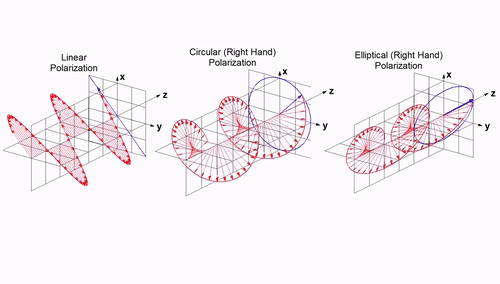•# On the polarization of electromagnetic waves

The property that the orientation and amplitude of electromagnetic wave electric field intensity change with time is called polarization in optics. If this change has a definite law, it is called polarized electromagnetic wave .

(hereinafter referred to as polarized wave)7 key points to know about “electromagnetic wave polarization” are:

1. Electromagnetic wave polarization refers to the property that the orientation and amplitude of electromagnetic wave electric field intensity change with time, which is called polarization in optics. If this change has a definite law, it is called polarized electromagnetic wave (hereinafter referred to as polarized wave). If the electric field intensity of a polarized electromagnetic wave is always oriented in a (transverse) plane perpendicular to the propagation direction, and the endpoint of its electric field vector moves along a closed track, this polarized electromagnetic wave is called a plane polarized wave. The sagittal trajectory of the electric field is called the polarization curve, and the polarization wave is named according to the shape of the polarization curve.

2. 2. For a single frequency plane polarized wave, the polarization curve is an ellipse (called polarization ellipse), so it is called elliptical polarized wave. Viewed from the propagation direction, if the rotation direction of the electric field vector is clockwise, which conforms to the right helix law, it is called right-handed polarized wave; If the rotation direction is counterclockwise and conforms to the left helix law, it is called left-handed polarized wave. According to the geometric parameters of the polarization ellipse (see the geometric parameters of the polarization ellipse), the elliptical polarization wave can be described quantitatively, that is, the axial ratio (the ratio of the long axis to the short axis), the polarization direction angle (the oblique angle of the long axis) and the rotation direction (right or left rotation). An elliptical polarized wave with an axial ratio equal to 1 is called a circular polarized wave, and its polarization curve is a circle, which can also be divided into right-handed or left-handed directions. At this time, the polarization direction angle is uncertain, and the oblique angle of the initial orientation of the electric field vector is replaced. The elliptical polarization wave whose axial ratio tends to infinity is called linear polarization wave. The orientation of its electric field vector is always on a straight line, and the oblique angle of this straight line is the polarization direction. At this time, the rotation direction loses its meaning and is replaced by the initial phase of the electric field intensity.

3. Any elliptical polarization wave can be decomposed into the sum of a right-handed circular polarization wave (represented by foot mark R) and a left-handed circular polarization wave (represented by foot mark L). If the linearly polarized wave is decomposed into two circularly polarized waves with opposite rotation directions, their amplitudes are equal and their initial orientation is symmetrical to that of the linearly polarized wave.

4. Any elliptical polarization wave can also be decomposed into the sum of two linearly polarized waves with orthogonal orientation. Generally, one of the linearly polarized waves is oriented in the horizontal plane (and perpendicular to the propagation direction), which is called the horizontally polarized wave (represented by foot mark h); The orientation of the other linearly polarized wave is simultaneously perpendicular to the orientation and propagation direction of the above horizontally polarized wave, which is called a vertically polarized wave (represented by foot mark V) (the electric field vector of the vertically polarized wave is oriented along the plumb line only when the propagation direction is in the horizontal plane). The electric field vectors of the two linearly polarized wave components have different amplitude sum and different initial phase sum.

5. The same elliptical polarization wave can be described quantitatively not only by the geometric parameters of the polarization ellipse, but also by the parameters between two counter rotating circular polarization components or two orthogonal linear polarization components. Polarization circle map is essentially the projection of isolines of various polarization parameters on the spherical surface on the equatorial plane. The antenna transmitting and receiving electromagnetic waves has definite polarization properties, which can be named according to the electromagnetic wave polarization in the strongest radiation direction when it is used as a transmitting antenna.

6. Generally, in order to achieve maximum power transmission between transmitting and receiving antennas, transmitting and receiving antennas with the same polarization properties should be used. This configuration condition is called polarization matching. Sometimes, in order to avoid the induction of a certain polarization wave, an antenna with orthogonal polarization properties is used, such as a vertical polarization antenna orthogonal to a horizontal polarization wave; The right-handed circularly polarized antenna is orthogonal to the left-handed circularly polarized wave. This configuration condition is called polarization isolation.

7. The potential isolation between two mutually orthogonal polarization waves can be applied to various dual polarization systems. For example, using a single antenna with dual polarization function to realize dual channel transmission or transceiver duplex; Two separate orthogonal polarization antennas are used to realize polarization diversity reception or stereoscopic observation (such as stereo film). In addition, in information detection systems such as remote sensing and radar target recognition, the polarization property of scattered waves can provide additional information besides amplitude and phase information.

Tel:(028) 84215383

Address:  No.24-2 Longtan Industrial Urban Park, Chenghua District, Chengdu, Sichuan, China

### E-mail:

Post time: May-06-2022
•#### Tel

##### Tel
•#### E-mail

##### E-mail
•